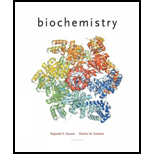Biochemistry
6th Edition
ISBN: 9781305577206
Author: Reginald H. Garrett, Charles M. Grisham
Publisher: Cengage LearningBiochemistry
Water: The Medium Of Life. 8P

# (a) To calculate: The approximate fractional concentrations of H 3 PO 4 at pH values of 0,2,4,6,8,10, and 12. Concept Introduction: Phosphoric acid has three H+ dissociations. H 3 PO 4 ⇌ H + + H 2 PO 4 − pK a 1 = 2.15 H 2 PO 4 − ⇌ H + + HPO 4 2 − pK a 2 = 7.20 HPO 4 2 − ⇌ H + + PO 4 3 − pK a 3 = 12.4 The Henderson-Hasselbalch equation is as follows: p H = p K a + log [ A − ] [ H A ]

QuestionChapter 2, Problem 8P
Interpretation Introduction

## (a)To calculate:The approximate fractional concentrations of H3PO4 at pH values of 0,2,4,6,8,10, and 12.Concept Introduction:Phosphoric acid has three H+ dissociations.  H3PO4⇌H++H2PO4−     pKa1=2.15H2PO4−⇌H++HPO42−     pKa2=7.20HPO42−⇌H++PO43−        pKa3=12.4The Henderson-Hasselbalch equation is as follows:  pH=pKa+log[A−][HA]

Expert Solution

### Explanation of Solution

Rearranging the Henderson-Hasselbalch equation as follows:

pH=pKa+log[ A ][HA][ A ][HA]=antilog(pHpKa)

At particular pH, [H2PO4-][H3PO4] = x, [HPO42-][H2PO4-] = y, [PO43-][HPO42-] = z

Fraction of any species at a particular pH is concentration of the species divided by the sum of the concentration of all species.

fH3PO4=[H3PO4][H3PO4]+[H2PO4-]+[HPO42-]+[PO43-]

We can rewrite the above equation as a function of x, y, and z.

fH3PO4=[ H 3 PO 4][ H 3 PO 4]+x[ H 3 PO 4]+xy[ H 3 PO 4]+xyz[ H 3 PO 4]fH3PO4=11+x+xy+xyz

We can manually calculate x, y, z and fractions of each species, but it is time consuming. Therefore, for efficiency we can calculate them using a spread sheet as follows:

 pH x y z 0 0.00707946 6.3096E-08 3.9811E-13 2 0.70794578 6.3096E-06 3.9811E-11 4 70.7945784 0.00063096 3.9811E-09 6 7079.45784 0.06309573 3.9811E-07 8 707945.784 6.30957344 3.9811E-05 10 70794578.4 630.957344 0.00398107 12 7079457844 63095.7344 0.39810717

Thus,

 pH Fraction of H3PO4 0 0.993 2 0.585 4 0.014 6 0.000 8 0.000 10 0.000 12 0.000
Interpretation Introduction

Expert Solution

### Explanation of Solution

Rearranging the Henderson-Hasselbalch equation as follows:

pH=pKa+log[ A ][HA][ A ][HA]=antilog(pHpKa)

At particular pH, [H2PO4-][H3PO4] = x, [HPO42-][H2PO4-] = y, [PO43-][HPO42-] = z

Fraction of any species at a particular pH is concentration of the species divided by the sum of the concentration of all species.

fH2PO4-=[H2PO4-][H3PO4]+[H2PO4-]+[HPO42-]+[PO43-]

We can rewrite the above equation as a function of x, y, and z.

fH2 PO4=[ H 2 PO 4 ] [ H 2 PO 4 ]x+[ H 2 PO 4 ]+y[ H 2 PO 4 ]+yz[ H 2 PO 4 ]fH2 PO4=11x+1+y+yz

We can manually calculate x, y, z and fractions of each species, but it is time consuming. Therefore, for efficiency we can calculate them using a spread sheet as follows:

 pH x y z 0 0.00707946 6.3096E-08 3.9811E-13 2 0.70794578 6.3096E-06 3.9811E-11 4 70.7945784 0.00063096 3.9811E-09 6 7079.45784 0.06309573 3.9811E-07 8 707945.784 6.30957344 3.9811E-05 10 70794578.4 630.957344 0.00398107 12 7079457844 63095.7344 0.39810717

Thus,

 pH Fraction of H2PO4- 0 0.007 2 0.415 4 0.985 6 0.941 8 0.137 10 0.002 12 0.000
Interpretation Introduction

Expert Solution

### Explanation of Solution

Rearranging the Henderson-Hasselbalch equation as follows:

pH=pKa+log[ A ][HA][ A ][HA]=antilog(pHpKa)

At particular pH, [H2PO4-][H3PO4] = x, [HPO42-][H2PO4-] = y, [PO43-][HPO42-] = z

Fraction of any species at a particular pH is concentration of the species divided by the sum of the concentration of all species.

fHPO42-=[HPO42-][H3PO4]+[H2PO4-]+[HPO42-]+[PO43-]

We can rewrite the above equation as a function of x, y, and z.

f HPO4 2=[ HPO 4 2] [ HPO 4 2 ] xy+ [ HPO 4 2 ]y+[ HPO 4 2]+z[ HPO 4 2]f HPO4 2=11 xy+1y+1+z

We can manually calculate x, y, z and fractions of each species, but it is time consuming. Therefore, for efficiency we can calculate them using a spread sheet as follows:

 pH x y z 0 0.00707946 6.3096E-08 3.9811E-13 2 0.70794578 6.3096E-06 3.9811E-11 4 70.7945784 0.00063096 3.9811E-09 6 7079.45784 0.06309573 3.9811E-07 8 707945.784 6.30957344 3.9811E-05 10 70794578.4 630.957344 0.00398107 12 7079457844 63095.7344 0.39810717

Thus,

 pH Fraction of HPO42- 0 0.000 2 0.000 4 0.001 6 0.059 8 0.863 10 0.994 12 0.715
Interpretation Introduction

Expert Solution

### Explanation of Solution

Rearranging the Henderson-Hasselbalch equation as follows:

pH=pKa+log[ A ][HA][ A ][HA]=antilog(pHpKa)

At particular pH, [H2PO4-][H3PO4] = x, [HPO42-][H2PO4-] = y, [PO43-][HPO42-] = z

Fraction of any species at a particular pH is concentration of the species divided by the sum of the concentration of all species.

fPO43-=[PO43-][H3PO4]+[H2PO4-]+[HPO42-]+[PO43-]

We can rewrite the above equation as a function of x, y, and z.

f PO4 3=[ PO 4 3] [ PO 4 3 ] xyz+ [ PO 4 3 ] yz+ [ PO 4 3 ]z+[ PO 4 3]f PO4 3=11 xyz+1 yz+1z+1

We can manually calculate x, y, z and fractions of each species, but it is time consuming. Therefore, for efficiency we can calculate them using a spread sheet as follows:

 pH x y z 0 0.00707946 6.3096E-08 3.9811E-13 2 0.70794578 6.3096E-06 3.9811E-11 4 70.7945784 0.00063096 3.9811E-09 6 7079.45784 0.06309573 3.9811E-07 8 707945.784 6.30957344 3.9811E-05 10 70794578.4 630.957344 0.00398107 12 7079457844 63095.7344 0.39810717

Thus,

 pH Fraction of PO43- 0 0.000 2 0.000 4 0.000 6 0.000 8 0.000 10 0.004 12 0.285

### Want to see more full solutions like this?

Subscribe now to access step-by-step solutions to millions of textbook problems written by subject matter experts!Biochemistry
6th Edition
ISBN: 9781305577206
Author: Reginald H. Garrett, Charles M. Grisham
Publisher: Cengage LearningBiochemistry
Water: The Medium Of Life. 8PHuman MovementWant to see more full solutions like this?
Subscribe now to access step-by-step solutions to millions of textbook problems written by subject matter experts.
Knowledge Booster••